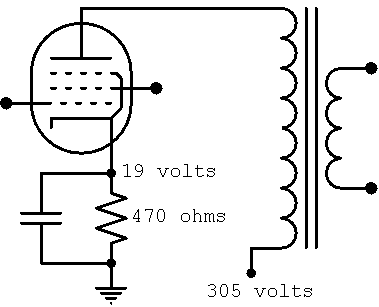According to Ohm's Law the current through the cathode resistor, flowing from the cathode to ground, is

19/470 = 40mA

Assuming the screen current is negligible, the plate current is also 40mA. This passes through the output transformer, flowing from the 305-volt plate supply to the plate, so the voltage across it is

(40mA)(50) = 2 volts

In a clockwise loop starting at the cathode, there is a voltage rise equal to the unknown plate voltage, then a 2-volt rise across the output transformer, then a 305-volt drop from the plate supply to ground, and then a 19-volt rise from ground to the cathode. According to Kirchhoff's Voltage Law, the sum of the rises is equal to the sum of the drops:

VP+2+19 = 305

which means

VP = 305-2-19 = 284

The 6V6GT plate voltage, measured from the plate to the cathode, is thus 284 volts.Guitar Amplifier Electronics: Basic Theory - a 21st-Century approach to understanding preamp, power amp, and power supply designFundamentals of Guitar Amplifier System Design - a structured methodology for crafting an awesome guitar amplifier!Guitar Amplifier Electronics: Circuit Simulation - a deep dive into SPICE tube amp modelingThe Fender Bassman 5F6-A - plus Marshall's Bluesbreaker and PlexiGeorg Simon Ohm - Imagine a world without Ohm's Law!